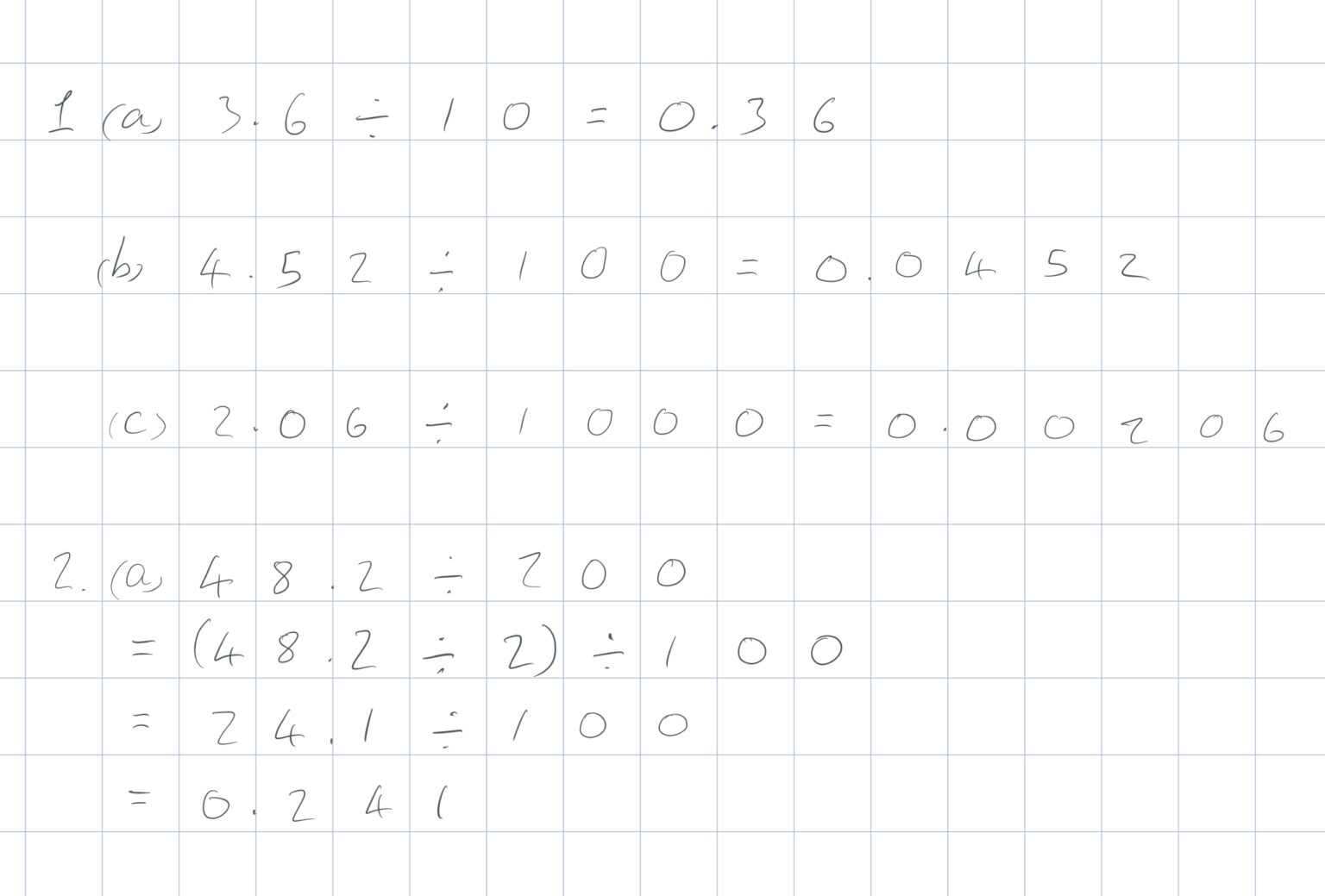To divide numbers by 10, move the numbers one place to the right.

To divide numbers by 100, move the numbers two places to the right.

To divide numbers by 1000, move the numbers three places to the right.

To divide numbers by multiples of 10, 100 or 1000, use two step division (see video from 1:20).

Remember: always keep the digits in the same order.